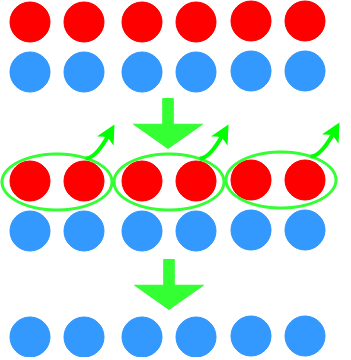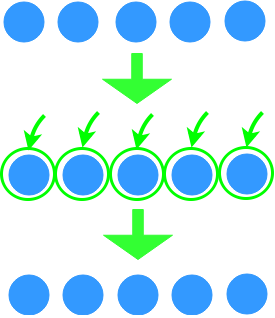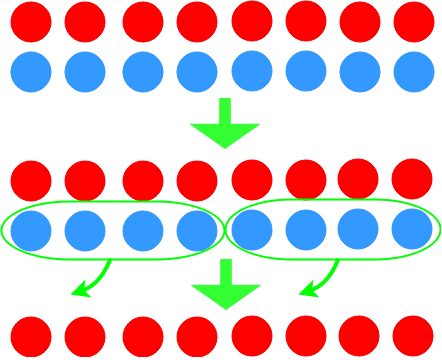Understanding integer multiplication

Understanding integer multiplication

In this section, we will learn the multiplication of positive and negative integers. At the beginning, diagrams will be used to help us getting familiar with the concept. We will then practice more with actual numbers.

Lessons

• Introduction
Introduction to integer multiplication

• 1.
Write the multiplication statement for each diagram.
a)b)c)• 2.
Solve.
a)
(-4)×(+6)

b)
(+7)×(+3)

c)
(-2)×(-9)

• 3.
Dave can do 15 sit-ups in one minute. How many sit-ups can he do in 8 minutes?

• 4.
A building has 8 stories above ground and 4 stories below ground. Each story has a height of 5m.
a)
What is the total height of the building above ground?

b)
What is the total depth of the building below ground?

• 5.
Mary spends $30 per week on meals for a year. Sally spends$125 per month on meals for a year. Who spends more money on meal, and by what amount?